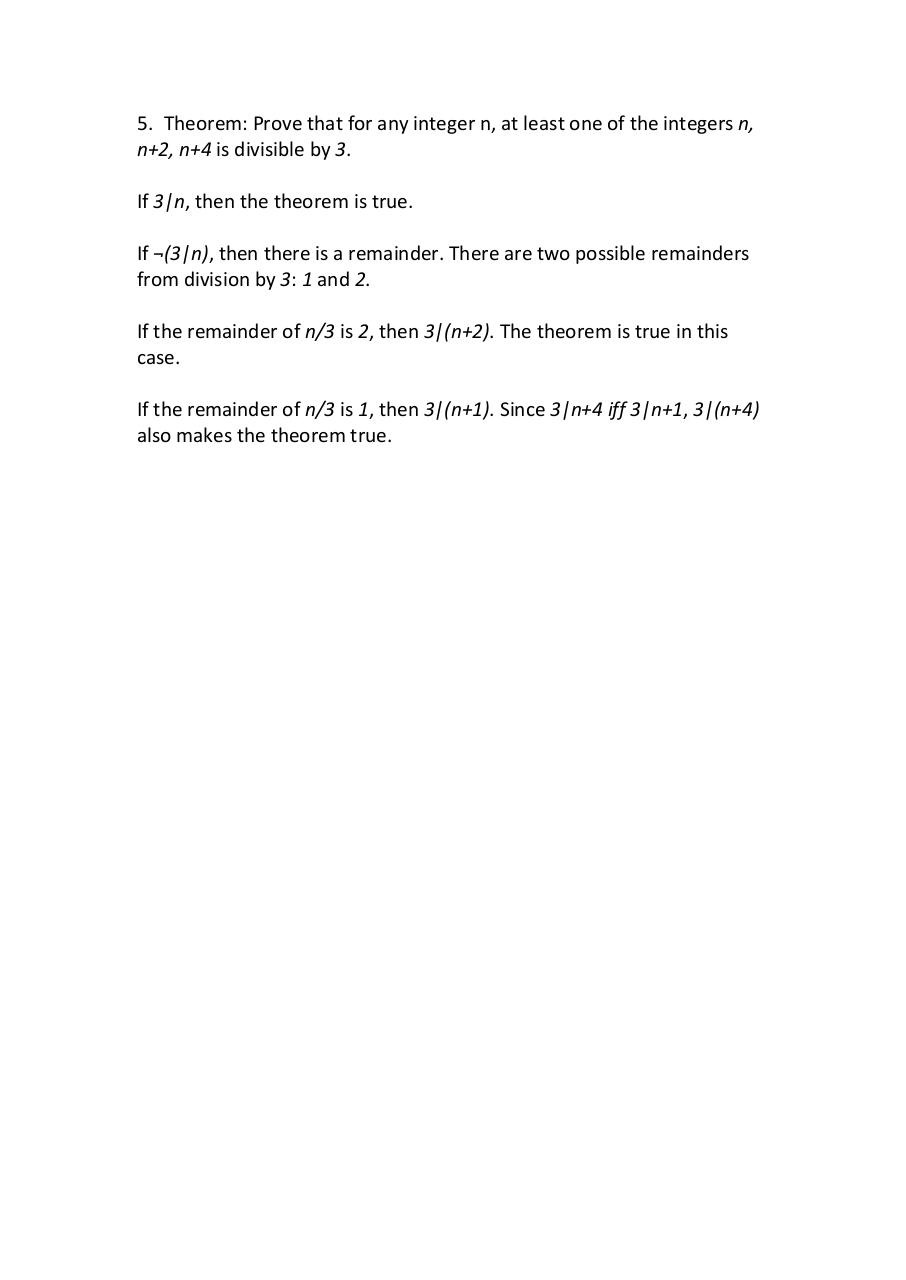# proof5 .pdf

### File information

Original filename: proof5.pdf
Author: vargh

This PDF 1.7 document has been generated by WPS Office / , and has been sent on pdf-archive.com on 28/08/2017 at 01:39, from IP address 108.218.x.x. The current document download page has been viewed 162 times.
File size: 45 KB (1 page).
Privacy: public file

proof5.pdf (PDF, 45 KB)

### Document preview

5. Theorem: Prove that for any integer n, at least one of the integers n,
n+2, n+4 is divisible by 3.
If 3|n, then the theorem is true.
If ¬(3|n), then there is a remainder. There are two possible remainders
from division by 3: 1 and 2.
If the remainder of n/3 is 2, then 3|(n+2). The theorem is true in this
case.
If the remainder of n/3 is 1, then 3|(n+1). Since 3|n+4 iff 3|n+1, 3|(n+4)
also makes the theorem true.#### HTML Code

Copy the following HTML code to share your document on a Website or Blog

#### QR Code### Related keywords